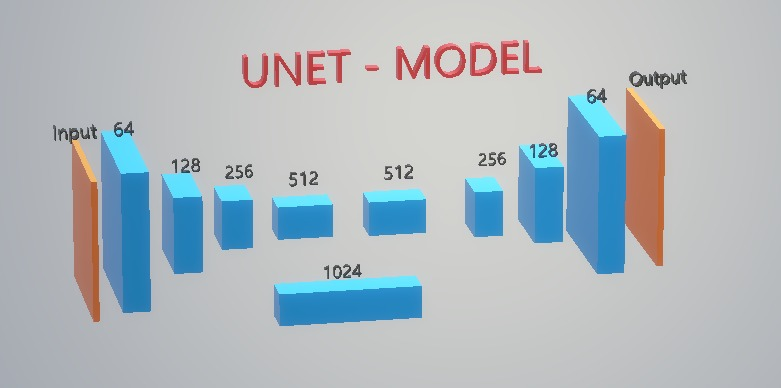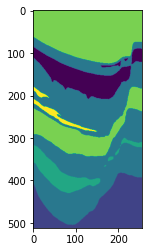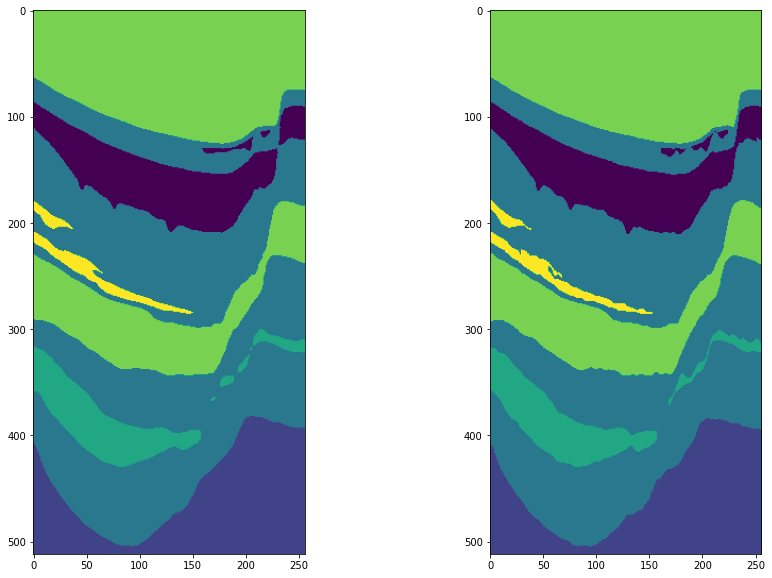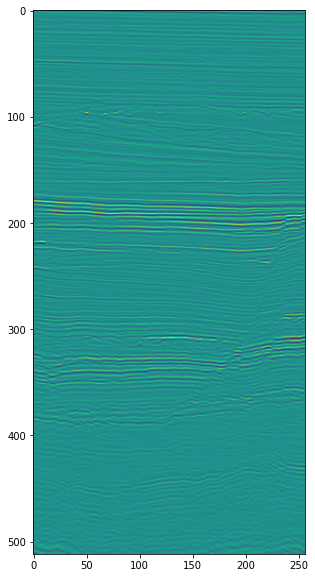Loading

# End to End solution that gives above 80% Accuracy

Updated the solution bit with some fine tuning and reshaping. The solution that able to give above 80% accuracy now.

Seismic facies identification refers to the interpretation of facies type from the seismic reflector information. The key elements used to determine seismic facies and depositional setting are bedform internal and external configuration/geometry, lateral continuity, amplitude, frequency, and interval velocity.

The classification of seismic facies is an important first step in exploration, prospecting, reservoir characterization, and field development.

Classification and interpretation of depositional facies from the chronostratigraphic units can provide initial indication as to whether the area of interest is a viable hydrocarbon system and merits additional research.

Furthermore, seismic facies classification can help in the approximation of grain size, sorting, mineralogy, porosity distribution, and permeability of the various deposition units.

When combined with open hole logging data, DHI, and advanced processing such as AVO, it is possible to estimate recovery and the potential for an economically viable prospect.

In modern seismic interpretation workflows, seismic facies classification is often automated or partially automated using computer algorithms, such as clustering and supervised learning.

Updated the solution bit with some fine tuning and reshaping. The solution that able to give above 80% accuracy now.

Colab Link of end 2 end solution - [Updated]

Above solution that handle ->

1. Loading the data
2. Slicing the data
3. Resize the data
4. Design UNet
5. Train the data
6. Predict the test data
7. Submission.npz

Few things we can tryout ->

1. Augumenting the data
2. Chunking and training insead of resizing the data
3. PSPNet, FPN ( other networks )4. Applying different filters

USEFULL NOTEBOOKS -

USEFULL LINKS -

1. https://github.com/frankkramer-lab/MIScnn [ 3D / 2D ]The open-source Python library MIScnn is an intuitive API allowing fast setup of image segmentation pipelines with state-of-the-art convolutional neural network and deep learning models in just a few lines of code.
2. https://github.com/microsoft/seismic-deeplearning
3. https://github.com/JesperDramsch/seismic-transfer-learning
4. https://github.com/wolny/pytorch-3dunet
5. https://github.com/goodok/fastai_sparse
6. https://github.com/arnab39/FewShot_GAN-Unet3D
7. https://github.com/black0017/MedicalZooPytorch
8. https://github.com/anindox8/Ensemble-of-Multi-Scale-CNN-for-3D-Brain-Segmentation
9. https://github.com/ShouYuqing/3D-UNet-for-Segmentation
10. https://github.com/fitushar/3DUnet_tensorflow2.0
11. https://neptune.ai/blog/image-segmentation-tips-and-tricks-from-kaggle-competitions
12. https://github.com/nikhilroxtomar/Deep-Residual-Unet
13. https://github.com/nikhilroxtomar/Polyp-Segmentation-using-UNET-in-TensorFlow-2.0
14. https://github.com/shivangi-aneja/Multi-Modal-Brain-Segmentation
15. https://github.com/ardamavi/3D-Medical-Segmentation-GAN

FEW RECENT PAPERS-

Thanks to the people who contribute that help us to learn more and also big thanks to aicrowd for these amazing challengesIn [ ]:
```import torch.nn as nn
import torch
import torch.nn.functional as F

# DoubleConv Class to perform two layer Convolution
class DoubleConv(nn.Module):
def __init__(self,in_ch,out_ch):
super(DoubleConv,self).__init__()
self.conv = nn.Sequential(
nn.Conv2d(in_ch,out_ch,3,padding=1),
nn.BatchNorm2d(out_ch),
nn.ReLU(inplace = True),
nn.Conv2d(out_ch,out_ch,3,padding=1),
nn.BatchNorm2d(out_ch),
nn.ReLU(inplace = True)
)
def forward(self,x):
return self.conv(x)
# Unter Class with shown architecture
class UNet(nn.Module):
def __init__(self,in_ch,out_ch):
super(UNet,self).__init__()
self.conv1 = DoubleConv(in_ch,64)
self.pool1 = nn.MaxPool2d(2)
self.conv2 = DoubleConv(64,128)
self.pool2 = nn.MaxPool2d(2)
self.conv3 = DoubleConv(128,256)
self.pool3 = nn.MaxPool2d(2)
self.conv4 = DoubleConv(256,512)
self.pool4 = nn.MaxPool2d(2)
self.conv5 = DoubleConv(512,1024)
self.up6 = nn.ConvTranspose2d(1024,512,2,stride=2)
self.conv6 = DoubleConv(1024,512)
self.up7 = nn.ConvTranspose2d(512,256,2,stride=2)
self.conv7 = DoubleConv(512,256)
self.up8 = nn.ConvTranspose2d(256,128,2,stride=2)
self.conv8 = DoubleConv(256,128)
self.up9 = nn.ConvTranspose2d(128,64,2,stride=2)
self.conv9 = DoubleConv(128,128)
self.conv9 = DoubleConv(128,64)
self.conv10 = nn.Conv2d(64,out_ch,1)

def forward(self,x):
c1 = self.conv1(x)
p1 = self.pool1(c1)
c2 = self.conv2(p1)
p2 = self.pool2(c2)
c3 = self.conv3(p2)
p3 = self.pool3(c3)
c4 = self.conv4(p3)
p4 = self.pool4(c4)
c5 = self.conv5(p4)
up_6 = self.up6(c5)
merge6 = torch.cat([up_6,c4],dim=1)
c6 = self.conv6(merge6)
up_7 = self.up7(c6)
merge7 = torch.cat([up_7,c3],dim=1)
c7 = self.conv7(merge7)
up_8 = self.up8(c7)
merge8 = torch.cat([up_8,c2],dim=1)
c8 = self.conv8(merge8)
up_9 = self.up9(c8)
merge9 = torch.cat([up_9,c1],dim=1)
c9 = self.conv9(merge9)
out = c9
c10 = self.conv10(c9)
out = c10
out = nn.Softmax()(c10)
return out
```

### We used U-Net model whose architecture is shown Below¶In [ ]:
```import torch
import torch.nn as nn

class DiceLoss(nn.Module):
def __init__(self):
super(DiceLoss, self).__init__()
def	forward(self, inputs, target):
N = target.size(0)
smooth = 1
input_flat = inputs.view(N, -1)
target_flat = target.view(N, -1)

intersection = input_flat * target_flat

loss = 2 * (intersection.sum(1) + smooth) / (input_flat.sum(1) + target_flat.sum(1) + smooth)
loss = 1 - loss.sum() / N

return loss

class MulticlassDiceLoss(nn.Module):
"""
requires one hot encoded target. Applies DiceLoss on each class iteratively.
requires input.shape[0:1] and target.shape[0:1] to be (N, C) where N is
batch size and C is number of classes
"""
def __init__(self):
super(MulticlassDiceLoss, self).__init__()

def forward(self, inputs, target, weights=None):

target = torch.nn.functional.one_hot(target.long()).permute(0,3,1,2)
inputs = torch.nn.functional.softmax(inputs,dim=1)

C = target.shape
# if weights is None:
# 	weights = torch.ones(C) #uniform weights for all classes

dice = DiceLoss()
totalLoss = 0

for i in range(C):
diceLoss = dice(inputs[:,i], target[:,i])
if weights is not None:
diceLoss *= weights[i]
totalLoss += diceLoss

return totalLoss
```
In [ ]:
```#!wget https://datasets.aicrowd.com/default/aicrowd-public-datasets/seamai-facies-challenge/v0.1/public/data_train.npz
#!wget https://datasets.aicrowd.com/default/aicrowd-public-datasets/seamai-facies-challenge/v0.1/public/data_test_1.npz
#!wget https://datasets.aicrowd.com/default/aicrowd-public-datasets/seamai-facies-challenge/v0.1/public/labels_train.npz
```
In [ ]:
```import time
import matplotlib.pyplot as plt
import numpy as np
import torch
from torch.utils.data import DataLoader, Dataset#
from torch import optim

import numpy as np
sei_patch = np.load('/content/data_train.npz')['data']
lab_patch = np.load('/content/labels_train.npz')['labels']

import cv2
from tqdm.notebook import tqdm
import datetime
from IPython.display import HTML
import cv2
```
In [ ]:
```sei_patch.shape , lab_patch.shape
```
Out[ ]:
`((1006, 782, 590), (1006, 782, 590))`
In [ ]:
```lab_patch[lab_patch==6] = 0
```
In [ ]:
```#np.unique(lab_patch)
```
In [ ]:
```training_img_data = []
training_label_data = []

#MAX_AMP = np.amax(sei_patch)*1.05 # I normalize over the max amp cos I wanna

# Define the X lines slices for training
for i in tqdm(range(0, sei_patch.shape)):
img = sei_patch[:, i, :]
label = lab_patch[:, i, :]

#img = img/MAX_AMP
#img = fast_glcm_entropy(img)
img = np.expand_dims(img, axis=2).astype('float32')
label = np.expand_dims(label, axis=2).astype('float32')

img = cv2.resize(img, (256, 512), interpolation=cv2.INTER_AREA)
label = cv2.resize(label, (256, 512), interpolation = cv2.INTER_NEAREST)
label = label.astype(int)
#img = np.clip(img, 0, 255)
#img = (img*255).astype(int)

#img = cv2.merge([img,img,img]) #we need 3 channels baby

#cv2.imwrite('/content/training_imgs/image_x_%03d.png' % i, img)
#cv2.imwrite('/content/training_labels/image_x_%03d.png' % i, label)

training_img_data.append(img)
training_label_data.append(label)

# Define the Y lines slices for training
for i in tqdm(range(0, sei_patch.shape)):
img = sei_patch[:, :, i]
label = lab_patch[:, :, i]

#img = img/MAX_AMP
#img = fast_glcm_entropy(img)
img = np.expand_dims(img, axis=2).astype('float32')
label = np.expand_dims(label, axis=2).astype('float32')

img = cv2.resize(img, (256, 512), interpolation=cv2.INTER_AREA)
label = cv2.resize(label, (256, 512), interpolation = cv2.INTER_NEAREST)
label = label.astype(int)

#img = np.clip(img, 0, 255)
#img = (img*255).astype(int)

#img = cv2.merge([img,img,img]) #we need 3 channels baby

#cv2.imwrite('/content/training_imgs/image_y_%03d.png' % i, img)
#cv2.imwrite('/content/training_labels/image_y_%03d.png' % i, label)
training_img_data.append(img)
training_label_data.append(label)
```

In [ ]:
```training_img_data = np.asarray(training_img_data)
training_label_data = np.asarray(training_label_data)
training_label_data = np.array(training_label_data,dtype=int)
training_img_data.shape, training_label_data.shape
```
Out[ ]:
`((1372, 512, 256), (1372, 512, 256))`
In [ ]:
```#np.unique(training_label_data)
```
In [ ]:
```class DataGenerator(Dataset):
def __init__(self, x_set, y_set):
self.x, self.y = x_set, y_set

def __len__(self):
return len(self.x)
def __getitem__(self, index):
batch_x = self.x[index]
batch_y = self.y[index]
return np.expand_dims(batch_x,axis=0), batch_y

e=1e-2

def accuracy(out, yb):
preds = torch.argmax(out, dim=1)
return (preds == yb).float().mean()

def train(model,optimizer,dataload,num_epochs,device):
acc_history  = []
loss_history = []
miou_history = []
for epoch in range(num_epochs):
print('Starting epoch {}/{}'.format(epoch+1, num_epochs))
print('-' * 10)
since = time.time()
dataset_size = len(dataload.dataset)
epoch_loss = 0
epoch_acc  = 0

for idx,(x, y) in enumerate(dataload):
optimizer.zero_grad()
inputs = x.to(device)
labels = y.to(device)
outputs = model(inputs)
criterion1 = MulticlassDiceLoss()
loss1 = criterion1(outputs,labels.long())
criterion2 = torch.nn.CrossEntropyLoss()
loss2 = criterion2(torch.log(outputs),labels.long())
loss = e*loss1+loss2
acc  = accuracy(outputs,labels)
loss.backward()
optimizer.step()

epoch_loss += loss.item()
epoch_acc+= acc
loss_history.append(loss.item())
acc_history.append(acc)
if (idx+1)%10==0:
print("%d/%d,train_loss:%0.3f,accuracy:%0.3f" % (idx+1, dataset_size // dataload.batch_size, loss.item(),acc))

time_elapsed = time.time() - since
all_epoch_loss=epoch_loss/len(dataload)
all_epoch_acc=epoch_acc/len(dataload)
print("epoch %d loss:%0.3f accuracy:%0.3f " % (epoch, all_epoch_loss,all_epoch_acc))
print('Training complete in {:.0f}m {:.0f}s'.format(time_elapsed // 60, time_elapsed % 60))

torch.save(model,"/content/model_0.pth")
return model,loss_history,acc_history
```
In [ ]:
```# from dice_loss import *
!CUDA_LAUNCH_BLOCKING=1
device = torch.device('cuda' if torch.cuda.is_available() else 'cpu')
model = UNet(1,6).to(device)
train_dataset = DataGenerator(x_set=training_img_data,y_set=training_label_data)
dataloader = DataLoader(train_dataset, batch_size=10, shuffle=True)
optimizer = optim.Adam(model.parameters(),lr=1e-2)
num_epochs=14
model_0,loss,acc=train(model,optimizer,dataloader,num_epochs,device)
```
```Starting epoch 1/14
----------
```
```/usr/local/lib/python3.6/dist-packages/ipykernel_launcher.py:68: UserWarning: Implicit dimension choice for softmax has been deprecated. Change the call to include dim=X as an argument.
```
```10/137,train_loss:1.159,accuracy:0.560
20/137,train_loss:0.906,accuracy:0.704
30/137,train_loss:0.714,accuracy:0.762
40/137,train_loss:0.624,accuracy:0.796
50/137,train_loss:0.544,accuracy:0.814
60/137,train_loss:0.471,accuracy:0.839
70/137,train_loss:0.394,accuracy:0.854
80/137,train_loss:0.392,accuracy:0.863
90/137,train_loss:0.354,accuracy:0.877
100/137,train_loss:0.314,accuracy:0.894
110/137,train_loss:0.282,accuracy:0.907
120/137,train_loss:0.261,accuracy:0.917
130/137,train_loss:0.318,accuracy:0.903
epoch 0 loss:0.552 accuracy:0.804
Training complete in 6m 13s
Starting epoch 2/14
----------
10/137,train_loss:0.285,accuracy:0.910
20/137,train_loss:0.252,accuracy:0.923
30/137,train_loss:0.222,accuracy:0.928
40/137,train_loss:0.210,accuracy:0.935
50/137,train_loss:0.211,accuracy:0.934
60/137,train_loss:0.177,accuracy:0.945
70/137,train_loss:0.186,accuracy:0.943
80/137,train_loss:0.180,accuracy:0.944
90/137,train_loss:0.163,accuracy:0.952
100/137,train_loss:0.173,accuracy:0.947
110/137,train_loss:0.156,accuracy:0.956
120/137,train_loss:0.153,accuracy:0.957
130/137,train_loss:0.173,accuracy:0.949
epoch 1 loss:0.203 accuracy:0.937
Training complete in 6m 14s
Starting epoch 3/14
----------
10/137,train_loss:0.158,accuracy:0.954
20/137,train_loss:0.173,accuracy:0.948
30/137,train_loss:0.158,accuracy:0.955
40/137,train_loss:0.146,accuracy:0.959
50/137,train_loss:0.158,accuracy:0.953
60/137,train_loss:0.143,accuracy:0.960
70/137,train_loss:0.131,accuracy:0.965
80/137,train_loss:0.134,accuracy:0.964
90/137,train_loss:0.139,accuracy:0.961
100/137,train_loss:0.159,accuracy:0.953
110/137,train_loss:0.128,accuracy:0.966
120/137,train_loss:0.141,accuracy:0.962
130/137,train_loss:0.130,accuracy:0.965
epoch 2 loss:0.149 accuracy:0.958
Training complete in 6m 15s
Starting epoch 4/14
----------
10/137,train_loss:0.138,accuracy:0.962
20/137,train_loss:0.141,accuracy:0.962
30/137,train_loss:0.129,accuracy:0.965
40/137,train_loss:0.119,accuracy:0.969
50/137,train_loss:0.118,accuracy:0.970
60/137,train_loss:0.131,accuracy:0.964
70/137,train_loss:0.120,accuracy:0.969
80/137,train_loss:0.120,accuracy:0.969
90/137,train_loss:0.118,accuracy:0.970
100/137,train_loss:0.117,accuracy:0.970
110/137,train_loss:0.109,accuracy:0.974
120/137,train_loss:0.109,accuracy:0.973
130/137,train_loss:0.112,accuracy:0.972
epoch 3 loss:0.124 accuracy:0.968
Training complete in 6m 17s
Starting epoch 5/14
----------
10/137,train_loss:0.109,accuracy:0.973
20/137,train_loss:0.112,accuracy:0.972
30/137,train_loss:0.117,accuracy:0.971
40/137,train_loss:0.110,accuracy:0.973
50/137,train_loss:0.119,accuracy:0.970
60/137,train_loss:0.132,accuracy:0.965
70/137,train_loss:0.118,accuracy:0.969
80/137,train_loss:0.121,accuracy:0.969
90/137,train_loss:0.112,accuracy:0.972
100/137,train_loss:0.107,accuracy:0.974
110/137,train_loss:0.099,accuracy:0.977
120/137,train_loss:0.112,accuracy:0.972
130/137,train_loss:0.101,accuracy:0.977
epoch 4 loss:0.114 accuracy:0.972
Training complete in 6m 16s
Starting epoch 6/14
----------
10/137,train_loss:0.103,accuracy:0.975
20/137,train_loss:0.112,accuracy:0.971
30/137,train_loss:0.099,accuracy:0.977
40/137,train_loss:0.096,accuracy:0.978
50/137,train_loss:0.094,accuracy:0.979
60/137,train_loss:0.108,accuracy:0.974
70/137,train_loss:0.113,accuracy:0.972
80/137,train_loss:0.105,accuracy:0.975
90/137,train_loss:0.098,accuracy:0.977
100/137,train_loss:0.095,accuracy:0.979
110/137,train_loss:0.095,accuracy:0.979
120/137,train_loss:0.091,accuracy:0.981
130/137,train_loss:0.094,accuracy:0.979
epoch 5 loss:0.102 accuracy:0.977
Training complete in 6m 16s
Starting epoch 7/14
----------
10/137,train_loss:0.103,accuracy:0.975
20/137,train_loss:0.094,accuracy:0.979
30/137,train_loss:0.093,accuracy:0.980
40/137,train_loss:0.092,accuracy:0.980
50/137,train_loss:0.089,accuracy:0.981
60/137,train_loss:0.094,accuracy:0.979
70/137,train_loss:0.090,accuracy:0.981
80/137,train_loss:0.086,accuracy:0.983
90/137,train_loss:0.086,accuracy:0.982
100/137,train_loss:0.087,accuracy:0.982
110/137,train_loss:0.087,accuracy:0.982
120/137,train_loss:0.091,accuracy:0.980
130/137,train_loss:0.108,accuracy:0.974
epoch 6 loss:0.095 accuracy:0.979
Training complete in 6m 16s
Starting epoch 8/14
----------
10/137,train_loss:0.119,accuracy:0.971
20/137,train_loss:0.108,accuracy:0.974
30/137,train_loss:0.100,accuracy:0.977
40/137,train_loss:0.093,accuracy:0.980
50/137,train_loss:0.089,accuracy:0.981
60/137,train_loss:0.088,accuracy:0.982
70/137,train_loss:0.089,accuracy:0.981
80/137,train_loss:0.088,accuracy:0.981
90/137,train_loss:0.085,accuracy:0.982
100/137,train_loss:0.085,accuracy:0.983
110/137,train_loss:0.083,accuracy:0.984
120/137,train_loss:0.086,accuracy:0.983
130/137,train_loss:0.085,accuracy:0.982
epoch 7 loss:0.093 accuracy:0.980
Training complete in 6m 17s
Starting epoch 9/14
----------
10/137,train_loss:0.089,accuracy:0.982
20/137,train_loss:0.098,accuracy:0.978
30/137,train_loss:0.097,accuracy:0.979
40/137,train_loss:0.091,accuracy:0.980
50/137,train_loss:0.090,accuracy:0.981
60/137,train_loss:0.088,accuracy:0.982
70/137,train_loss:0.080,accuracy:0.985
80/137,train_loss:0.081,accuracy:0.984
90/137,train_loss:0.082,accuracy:0.984
100/137,train_loss:0.083,accuracy:0.984
110/137,train_loss:0.079,accuracy:0.985
120/137,train_loss:0.083,accuracy:0.984
130/137,train_loss:0.079,accuracy:0.985
epoch 8 loss:0.088 accuracy:0.982
Training complete in 6m 16s
Starting epoch 10/14
----------
10/137,train_loss:0.079,accuracy:0.985
20/137,train_loss:0.080,accuracy:0.985
30/137,train_loss:0.079,accuracy:0.985
40/137,train_loss:0.079,accuracy:0.985
50/137,train_loss:0.077,accuracy:0.986
60/137,train_loss:0.078,accuracy:0.986
70/137,train_loss:0.079,accuracy:0.985
80/137,train_loss:0.075,accuracy:0.987
90/137,train_loss:0.080,accuracy:0.985
100/137,train_loss:0.076,accuracy:0.987
110/137,train_loss:0.078,accuracy:0.985
120/137,train_loss:0.076,accuracy:0.987
130/137,train_loss:0.119,accuracy:0.971
epoch 9 loss:0.084 accuracy:0.984
Training complete in 6m 17s
Starting epoch 11/14
----------
10/137,train_loss:0.111,accuracy:0.974
20/137,train_loss:0.101,accuracy:0.976
30/137,train_loss:0.089,accuracy:0.981
40/137,train_loss:0.089,accuracy:0.981
50/137,train_loss:0.080,accuracy:0.985
60/137,train_loss:0.115,accuracy:0.970
70/137,train_loss:0.095,accuracy:0.979
80/137,train_loss:0.089,accuracy:0.981
90/137,train_loss:0.088,accuracy:0.983
100/137,train_loss:0.080,accuracy:0.985
110/137,train_loss:0.078,accuracy:0.986
120/137,train_loss:0.079,accuracy:0.985
130/137,train_loss:0.078,accuracy:0.985
epoch 10 loss:0.091 accuracy:0.981
Training complete in 6m 15s
Starting epoch 12/14
----------
10/137,train_loss:0.077,accuracy:0.986
20/137,train_loss:0.101,accuracy:0.977
30/137,train_loss:0.091,accuracy:0.981
40/137,train_loss:0.087,accuracy:0.982
50/137,train_loss:0.086,accuracy:0.982
60/137,train_loss:0.077,accuracy:0.986
70/137,train_loss:0.079,accuracy:0.985
80/137,train_loss:0.074,accuracy:0.987
90/137,train_loss:0.074,accuracy:0.987
100/137,train_loss:0.076,accuracy:0.986
110/137,train_loss:0.080,accuracy:0.985
120/137,train_loss:0.074,accuracy:0.987
130/137,train_loss:0.074,accuracy:0.987
epoch 11 loss:0.082 accuracy:0.985
Training complete in 6m 14s
Starting epoch 13/14
----------
10/137,train_loss:0.075,accuracy:0.987
20/137,train_loss:0.072,accuracy:0.988
30/137,train_loss:0.072,accuracy:0.988
40/137,train_loss:0.072,accuracy:0.988
50/137,train_loss:0.073,accuracy:0.988
60/137,train_loss:0.073,accuracy:0.987
70/137,train_loss:0.072,accuracy:0.988
80/137,train_loss:0.073,accuracy:0.988
90/137,train_loss:0.071,accuracy:0.989
100/137,train_loss:0.088,accuracy:0.982
110/137,train_loss:0.091,accuracy:0.981
120/137,train_loss:0.086,accuracy:0.982
130/137,train_loss:0.081,accuracy:0.984
epoch 12 loss:0.079 accuracy:0.986
Training complete in 6m 15s
Starting epoch 14/14
----------
10/137,train_loss:0.076,accuracy:0.986
20/137,train_loss:0.079,accuracy:0.985
30/137,train_loss:0.073,accuracy:0.988
40/137,train_loss:0.072,accuracy:0.988
50/137,train_loss:0.086,accuracy:0.983
60/137,train_loss:0.079,accuracy:0.986
70/137,train_loss:0.076,accuracy:0.987
80/137,train_loss:0.077,accuracy:0.986
90/137,train_loss:0.073,accuracy:0.988
100/137,train_loss:0.072,accuracy:0.988
110/137,train_loss:0.070,accuracy:0.989
120/137,train_loss:0.070,accuracy:0.989
130/137,train_loss:0.069,accuracy:0.989
epoch 13 loss:0.077 accuracy:0.987
Training complete in 6m 16s
```
In [ ]:
```def seisfacies_predict(section,patch_size=256,overlap=0,onehot=0):
m1,m2 = section.shape
os    = overlap
n1,n2 = 512,patch_size
c1 = int(np.round((m1+os)/(n1-os)+0.5))
c2 = int(np.round((m2+os)/(n2-os)+0.5))
p1 = (n1-os)*c1+os
p2 = (n2-os)*c2+os

gp = np.zeros((p1,p2),dtype=np.single)
gy = np.zeros((6,p1,p2),dtype=np.single)
gs = np.zeros((n1,n2),dtype=np.single)

gp[0:m1,0:m2]=section

for k1 in range(c1):
for k2 in range(c2):
b1 = k1*n1-k1*os
e1 = b1+n1
b2 = k2*n2-k2*os
e2 = b2+n2
#predict
gs[:,:]=gp[b1:e1,b2:e2]
x=gs.reshape(1,1,512,256)
Y_patch= model(torch.from_numpy(x)).squeeze()
p=F.softmax(Y_patch, dim=0).detach().numpy()
gy[:,b1:e1,b2:e2]= gy[:,b1:e1,b2:e2]+p

gy_onehot = gy[:,0:m1,0:m2]
#onehot2label
gy_label =np.argmax(gy_onehot,axis=0)

if onehot==0:
return gy_label
if onehot==1:
return gy_label,gy_onehot
```
In [ ]:
```#plt.imshow(training_label_data)
```
In [ ]:
```model = torch.load("model_0.pth",map_location='cpu')
gy_label,gy_onehot=seisfacies_predict(training_img_data,onehot=1)
plt.imshow(gy_label)
```
```/usr/local/lib/python3.6/dist-packages/ipykernel_launcher.py:68: UserWarning: Implicit dimension choice for softmax has been deprecated. Change the call to include dim=X as an argument.
```
Out[ ]:
`<matplotlib.image.AxesImage at 0x7f1c5c6405f8>`In [ ]:
```plt.rcParams["figure.figsize"] = (15, 10)
f, axarr = plt.subplots(1,2)
axarr.imshow(gy_label)
axarr.imshow(training_label_data)
```
Out[ ]:
`<matplotlib.image.AxesImage at 0x7f1c5c139d30>`In [ ]:
```training_label_data
```
Out[ ]:
```array([[4, 4, 4, ..., 4, 4, 4],
[4, 4, 4, ..., 4, 4, 4],
[4, 4, 4, ..., 4, 4, 4],
...,
[1, 1, 1, ..., 1, 1, 1],
[1, 1, 1, ..., 1, 1, 1],
[1, 1, 1, ..., 1, 1, 1]])```
In [ ]:
```gy_label
```
Out[ ]:
```array([[4, 4, 4, ..., 4, 4, 4],
[4, 4, 4, ..., 4, 4, 4],
[4, 4, 4, ..., 4, 4, 4],
...,
[1, 1, 1, ..., 1, 1, 1],
[1, 1, 1, ..., 1, 1, 1],
[1, 1, 1, ..., 1, 1, 1]])```
In [ ]:
```lab_patch[:,:,420]
```
Out[ ]:
```array([[4, 4, 4, ..., 4, 4, 4],
[4, 4, 4, ..., 4, 4, 4],
[4, 4, 4, ..., 4, 4, 4],
...,
[1, 1, 1, ..., 1, 1, 1],
[1, 1, 1, ..., 1, 1, 1],
[1, 1, 1, ..., 1, 1, 1]], dtype=int8)```
In [ ]:
```print('The amount of labels on the training label set was: {}.'.format(np.unique(gy_label)), 'The amount of labels on the predicted example is: {}'.format(np.unique(training_label_data)))
```
```The amount of labels on the training label set was: [0 1 2 3 4 5]. The amount of labels on the predicted example is: [0 1 2 3 4 5]
```
In [ ]:
```#from google.colab import drive
#drive.mount('/content/drive')
```
In [ ]:
```#import torch
#model = torch.load("model_0.pth",map_location='cpu')
#torch.save(model,"/content/drive/My Drive/Colab Notebooks/TestmodelNew11.pth")
```
In [ ]:
```test_seismic = np.load('/content/data_test_1.npz')['data']
```
In [ ]:
```test_seismic.shape
```
Out[ ]:
`(1006, 782, 251)`
In [ ]:
```testing_img_data = []
for i in tqdm(range(0, test_seismic.shape)):
img = test_seismic[:,i,:]
#img = img/MAX_AMP
img = np.expand_dims(img, axis=2).astype('float32')
img = cv2.resize(img, (256, 512))
testing_img_data.append(img)

for i in tqdm(range(0, test_seismic.shape)):
img = test_seismic[:,:,i]
#img = img/MAX_AMP
img = np.expand_dims(img, axis=2).astype('float32')
img = cv2.resize(img, (256, 512))
testing_img_data.append(img)

testing_img_data = np.asarray(testing_img_data)
```

In [ ]:
```testing_img_data.shape
```
Out[ ]:
`(1033, 512, 256)`
In [ ]:
```plt.imshow(testing_img_data)
```
Out[ ]:
`<matplotlib.image.AxesImage at 0x7f1c5b862198>`In [ ]:
```%%time
preds = []
for i in tqdm(range(0, test_seismic.shape)):
gy_label,gy_onehot=seisfacies_predict(testing_img_data[i],onehot=1)
#label = gy_label
#label = np.expand_dims(label, axis=2).astype('float32')
#label = cv2.resize(label, (251,1006))
preds.append(gy_label)
```
```/usr/local/lib/python3.6/dist-packages/ipykernel_launcher.py:68: UserWarning: Implicit dimension choice for softmax has been deprecated. Change the call to include dim=X as an argument.
```
```CPU times: user 3h 13min 7s, sys: 1min 33s, total: 3h 14min 41s
Wall time: 3h 14min 42s
```
In [ ]:
```preds = np.asarray(preds)
```
In [ ]:
```def reshaping(data, out_shape):
output_labels = []
for i in data:
img = np.expand_dims(i, axis=2).astype('float32')
img = cv2.resize(img, out_shape)
output_labels.append(img)
output_labels = np.asarray(output_labels)
output_labels = output_labels.astype(int)
return np.swapaxes(output_labels,0,1)
```
In [ ]:
```preds = reshaping(preds, (251,1006))
```
In [ ]:
```preds[preds == 0] = 6
```
In [ ]:
```#print(preds.shape, np.unique(preds))
```
In [ ]:
```np.savez_compressed(
"/content/prediction.npz",
prediction=preds
)
```
In [ ]:
```preds
```
Out[ ]:
```array([[[4, 4, 4, ..., 4, 4, 4],
[4, 4, 4, ..., 4, 4, 4],
[4, 4, 4, ..., 4, 4, 4],
...,
[4, 4, 4, ..., 4, 4, 4],
[4, 4, 4, ..., 4, 4, 4],
[4, 4, 4, ..., 4, 4, 4]],

[[4, 4, 4, ..., 4, 4, 4],
[4, 4, 4, ..., 4, 4, 4],
[4, 4, 4, ..., 4, 4, 4],
...,
[4, 4, 4, ..., 4, 4, 4],
[4, 4, 4, ..., 4, 4, 4],
[4, 4, 4, ..., 4, 4, 4]],

[[4, 4, 4, ..., 4, 4, 4],
[4, 4, 4, ..., 4, 4, 4],
[4, 4, 4, ..., 4, 4, 4],
...,
[4, 4, 4, ..., 4, 4, 4],
[4, 4, 4, ..., 4, 4, 4],
[4, 4, 4, ..., 4, 4, 4]],

...,

[[1, 1, 1, ..., 1, 1, 1],
[1, 1, 1, ..., 1, 1, 1],
[1, 1, 1, ..., 1, 1, 1],
...,
[1, 1, 1, ..., 1, 1, 1],
[1, 1, 1, ..., 1, 1, 1],
[1, 1, 1, ..., 1, 1, 1]],

[[1, 1, 1, ..., 1, 1, 1],
[1, 1, 1, ..., 1, 1, 1],
[1, 1, 1, ..., 1, 1, 1],
...,
[1, 1, 1, ..., 1, 1, 1],
[1, 1, 1, ..., 1, 1, 1],
[1, 1, 1, ..., 1, 1, 1]],

[[1, 1, 1, ..., 1, 1, 1],
[1, 1, 1, ..., 1, 1, 1],
[1, 1, 1, ..., 1, 1, 1],
...,
[1, 1, 1, ..., 1, 1, 1],
[1, 1, 1, ..., 1, 1, 1],
[1, 1, 1, ..., 1, 1, 1]]])```
In [ ]:
```
```

Liked by#### Comments

You must login before you can post a comment.By
pyanishjain
3 months ago

seems amazing thanksBy
umair_ahmed
3 months ago

Amazing bro. It gives a lot of info.